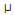# Electronics - Analog and Digital Converters

### Exercise :: Analog and Digital Converters - General Questions

6.

The difference between analog voltage represented by two adjacent digital codes, or the analog step size, is the:

 A. quantization B. accuracy C. resolution D. monotonicity

Explanation:

No answer description available for this question. Let us discuss.

7.

The primary disadvantage of the flash analog-to digital converter (ADC) is that:

 A. it requires the input voltage to be applied to the inputs simultaneously B. a long conversion time is required C. a large number of output lines is required to simultaneously decode the input voltage D. a large number of comparators is required to represent a reasonable sized binary number

Explanation:

No answer description available for this question. Let us discuss.

8.

A binary-weighted digital-to-analog converter has a feedback resistor, Rf, of 12 k. If 50A of current is through the resistor, the voltage out of the circuit is:

 A. 0.6 V B. –0.6 V C. 0.1 V D. –0.1 V

Explanation:

No answer description available for this question. Let us discuss.

9.

What is the major advantage of the R/2R ladder digital-to-analog (DAC), as compared to a binary-weighted digital-to-analog DAC converter?

 A. It only uses two different resistor values. B. It has fewer parts for the same number of inputs. C. Its operation is much easier to analyze. D. The virtual ground is eliminated and the circuit is therefore easier to understand and troubleshoot.

Explanation:

No answer description available for this question. Let us discuss.

10.

The resolution of a 0–5 V 6-bit digital-to-analog converter (DAC) is:

 A. 63% B. 64% C. 1.56% D. 15.6%